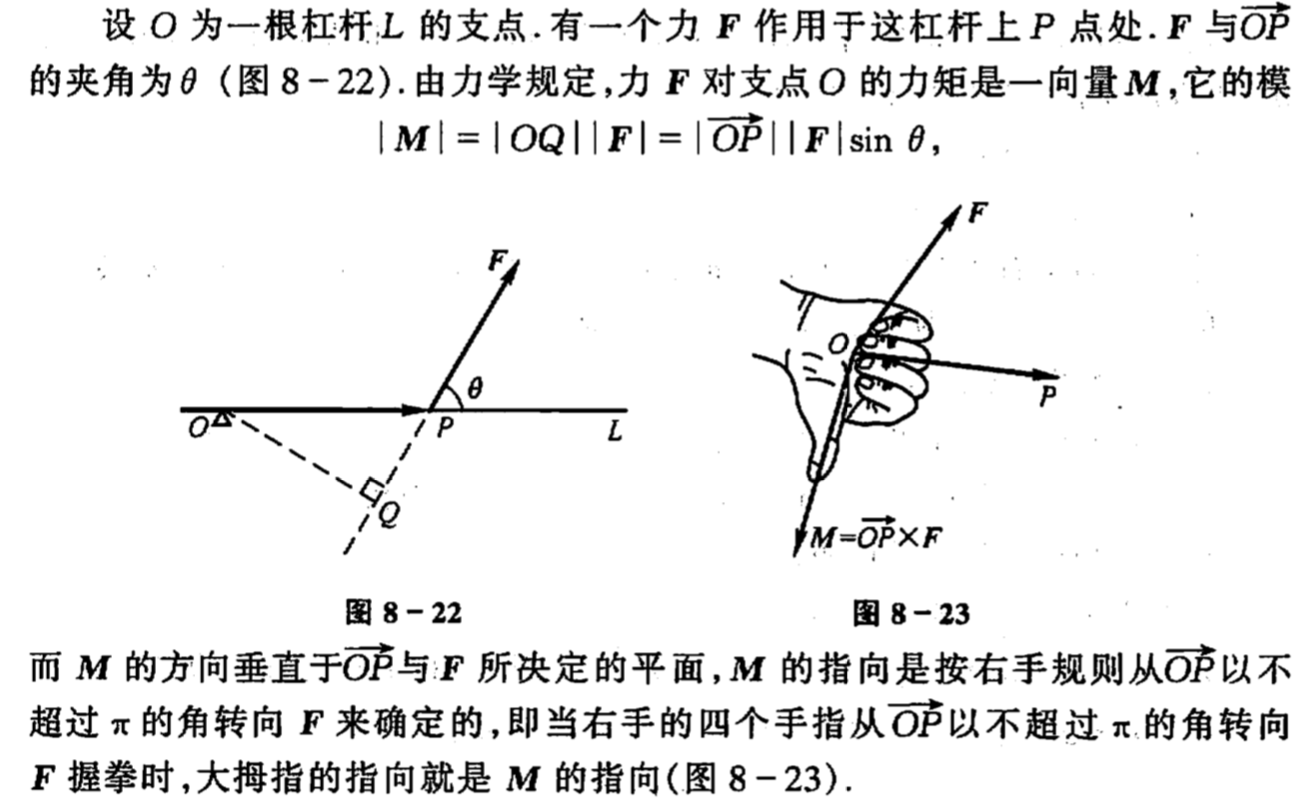• 一.向量及其线性运算 1.向量：客观世界有这样一类量，他们既有大 小，又有方向。...2.自由向量：与起点无关的向量，只考虑向量的大小与方向。 3.如果两个向量的大小相等且方向相同，就说向量a和向量b是相等的。 4. ...
一.向量及其线性运算
1.向量：客观世界有这样一类量，他们既有大       小，又有方向。
2.自由向量：与起点无关的向量，只考虑向量的大小与方向。
3.如果两个向量的大小相等且方向相同，就说向量a和向量b是相等的。
4. 向量的大小叫做  向量的模，模等于1的向量叫做单位向量，模等于0的向量叫做零向量  ，零向量与任何向量垂直，与任何向量平行。
5. 向量的加减法：三角形法则，平行四边形法则
6. 设a为一向量，与a的模大小相等而方向相反的向量叫做a的负向量，记作-a
7. 向量与数的乘法：结合律，分配律
8. 非零向量除以它的模的结果是一个与原向量同方向的单位向量
9. 定理：设向量a不等于0，则向量b平行于a的充分必要条件是：存在唯一的实数x，使b=xa
10.方向角：非零向量r与三条坐标轴的夹角A，B，C称为向量r的方向角，cosA，cosB,cosC称为向量r的方向余弦，cosAcosA+cosBcosB+cosC*cosC=1
设r=（x，y，z），则方向余弦cosA=x/|r|。。。
11.向量的投影具有与坐标相同的性质：
（1）Prja=|a|cosA(其中A为向量a与u轴的夹角)
（2）Prj（a+b）=Prja+Prjb
（3）Prj（xa）=xPrja二.数量积、向量积、混合积
1.数量积：a﹡b=|a| |b| cosA(其中A为a与b的夹角)
（两向量的数量积等于其中一个向量的模和另一个向量在这个向        量的方向上的投影的乘积）
2. 由数量积的定义可以推的：
（1）a﹡a=|a|﹡|a|
（2）对于两个非零向量a、b，如果a﹡b=0，那么a、b垂直，反之，如果a、b垂直，那么a﹡b=0；
3.数量积符合交换律，分配律，结合律
4.向量积：c=a×b=|a| |b| sinA（A为两向量之间的夹角）
5.向量积的定义推的：
（1）a×a=0
（2）对于两个非零向量a、b，如果a×b=0，那么a//b;反之，如果a//b,那么a×b=0
6.向量积符合下列运算规律
（1）a×b=-b×a
（2）分配律：（a+b）×c=a×c+b×c
（3）结合律
7.向量积的坐标表示
a=ax i+ay j+az k;     b=bx i+by j+bz k
a×b=（aybz-azby）i+(azbx-axbz) j+(axby-aybx)k
用行列式表达：
|    i                    j                  k|
|     ax              ay              az|= a×b
|     bx               by              bz|
8.混合积
（a×b）﹡c    记作 [ abc ]=|a×b| |c| cosA三.平面及其方程
1.  如果一个非零向量垂直于一平面，这向量就叫做该平面的法线向量
2.平面上的任一向量均与该平面上的法线向量垂直
3.设法线向量n=（A,B,C）  平面上的一点M=（x0，y0，z0）
（1）点法式方程：A（x-x0）+B（y-y0）+C(z-z0)=0
（2）一般方程：Ax+By+Cz+D=0
【x,y,z的系数就是该平面的一个法线向量n的做坐标，即n=（A,B,C）】
D=0，方程表示通过一个原点的平面
A=0，法线向量n=（0,B,C）垂直于x轴，方程表示一个平行于x轴的平面
同理B=0,C=0
A=B=0，方程表示一个平行于xOy面的平面，同理B=C=0,A=C=0
（3）截距式方程:x/a+y/b+z/c=0
【a，b，c依此叫做平面在x，y，z轴上的截距】

两平面之间的夹角
两平面的法线向量夹角（通常指锐角或直角）称为两平面的夹角
设两平面的法线向量为n1=（A1,B1,C1）,n2=（A2,B2,C2）
cos=|A1A2+B1B2+C1C2| /(根号(A1A1+B1B1+C1C1))×(根号(A2A2+B2B2+C2C2)）

5.点到平面的距离
点P（x0，y0，z0）               平面 Ax+By+Cz+D=0
d=|Ax0+By0+Cz0+D| /（根号下(A﹡A+B﹡B+C﹡C)）四.空间直线及方程
1.如果一个非零向量平行于已知向量,那么这个向量就叫做这条直线的方向向量
2.直线的表达式：
空间直线的一般方程：{A1 x+B1 y+C1 z+D1=0，A2 x+B2 y+C2 z+D2=0}
【两个平面方程的联立】
直线的对称式方程 / 点向式方程：(x-x0)/m=(y-y0)/n=(z-z0)/p=t
【方向向量s=（m,n,p），点M0=（x0,y0.z0)】
直线的参数方程：x=x0+mt；    y=y0+nt；   z=z0+pt
3.两直线的夹角
两直线的方向向量的夹角（通常指锐角或直角）叫做两直线的夹角
两直线互相垂直：m1m2+n1n2+p1p2=0
两直线相互平行或重合：m1/m2=n1/n2=p1/p2
4.直线与平面的夹角
当直线与平面不垂直时，直线与它在平面上的投影直线的夹角称为直线与平面的夹角（0~90）
直线方向向量s=(m,n,p)        ，平面的法线向量n=(A,B,C）
sin=|Am+Bn+Cp| /(根号(A﹡A+B﹡B+C﹡C))×(根号(m﹡m+n﹡n+p﹡p)）
直线与平面垂直：A/m=B/n=C/p
直线与平面平行或重合：Am+Bn+Cp=0
!展开全文• 1.1 一般在数学上只研究起点无关的向量（自由向量），即只考虑向量的大小方向。 1.2 向量的大小叫做向量的模。 1.3 非零向量r⃗三条坐标轴的夹角α,β,γ称为向量r⃗的方向角。非零向量\vec r三条坐标轴的...
1，向量的概念
1.1 一般在数学上只研究与起点无关的向量（自由向量），即只考虑向量的大小和方向。
1.2 向量的大小叫做向量的模。
1.3 $非零向量\vec r与三条坐标轴的夹角\alpha,\beta,\gamma称为向量\vec r的方向角。$
1.4 $\cos\alpha,\cos\beta,\cos\gamma称为向量\vec r的方向余弦。$
$以向量\vec r的方向余弦为坐标的向量就是与\vec r同方向的单位向量\vec e.$
2，基本运算
2.1 数量积（结果为一个数值）
$\vec a \times\vec b=|\vec a|\times|\vec b|\times\cos\theta$
2.2 向量积（结果为一个向量）
$\vec a \times\vec b=\vec c，$
其中$\vec c的模为|\vec c|=|\vec a|\times|\vec b|\times\sin\theta.$
方向判断方法如下：向量积与数量积不同点在于它不满足交换律：
$\vec a \times\vec b=-\vec b \times\vec a$.
（向量积除了不满足交换律，其它的性质和数量积一样，包括进行极限运算时。）
向量积可以写成行列式的形式：
$\vec a=(a_x,a_y,a_z), \vec b=(b_x,b_y,b_z)$，则
$\vec a \times\vec b= \begin{vmatrix} \vec i&\vec j&\vec k \\ a_x&a_y&a_z \\ b_x&b_y&b_z \\ \end{vmatrix}$
2.3 混合积
$先把向量\vec a 和\vec b作向量积，再与向量\vec c作数量积。$
$[\vec a\vec b\vec c]=(\vec a \times\vec b) \vec c= \begin{vmatrix} a_x&a_y&a_z \\ b_x&b_y&b_z \\ c_x&c_y&c_z \\ \end{vmatrix}$
混合积的几何意义：$它是这样一个数，它的绝对值表示以向量\vec a,\vec b,\vec c为棱的平行六面体的体积。$
3，曲面及其方程
3.1 在空间解析几何中，任何曲面都可以看做点的几何轨迹。在这样的意义下，如果曲面S与三元方程:
F(x,y,z)=0有下述关系：
（1），曲面S上任一点的坐标都满足方程（1）；
（2），不在曲面S上的点的坐标都不满足方程（1）；
那么，方程（1）就叫做曲面S的方程，而曲面S就叫做方程(1)的图形。
3.2 空间曲面的参数方程。
通常是含有两个参数的方程，形式如下：
$\begin{cases} x=x(s,t), \\ y=y(s,t), \\ z=z(s,t). \\ \end{cases}$
4，平面及其方程
4.1 点法式方程
$已知平面的法向量\vec n=(A,B,C)和平面上一点M_0(x_0,y_0,z_0)，则平面的方程为$
$A(x-x_0)+B(y-y_0)+C(z-z_0)=0$。
4.2 一般方程
三元一次方程对应着一个平面：
$Ax+By+Cz+D=0$。
5，空间直线及其方程
5.1 一般方程
可以理解为两个平面相交得到的直线
$\begin{cases} A_1x+B_1y+C_1z+D_1=0, \\ A_2x+B_2y+C_2z+D_2=0. \\ \end{cases}$
5.2 点向式方程（对称式方程）
$已知直线的方向向量\vec s=(m,n,p)和平面上一点M_0(x_0,y_0,z_0)，则直线的方程为$
$\frac{x-x_0}{m}=\frac{y-y_0}{n}=\frac{z-z_0}{p}$（如果两向量平行，那么它们的各个坐标之比相等）。
5.3 参数方程
如果令$\frac{x-x_0}{m}=\frac{y-y_0}{n}=\frac{z-z_0}{p}=t$，则
$\begin{cases} x=x_0+mt, \\ y=y_0+nt, \\ z=z_0+pt. \\ \end{cases}$


展开全文空间解析几何
• 2.向量的大小叫做向量的模。模等于1的向量叫做单位向量。模等于0的向量叫做零向量，零向量的方向可以看作是任意的。 3.向量相加有三角形法则和平行四边形法则。向量的加法满足（1）交换律和（2）结合律。 4.设a为...
1.数学上只研究与起点无关的向量，并称这种向量为自由向量（简称向量）。
2.向量的大小叫做向量的模。模等于1的向量叫做单位向量。模等于0的向量叫做零向量，零向量的方向可以看作是任意的。
3.向量相加有三角形法则和平行四边形法则。向量的加法满足（1）交换律和（2）结合律。
4.设a为一向量，与a的模相同而方向相反的向量叫做a的负向量，记作-a。规定两个向量b与a的差：b - a = b + (-a)。
5.向量与数的乘法满足（1）结合律和（2）分配律。
6.定理一：设向量a≠0，那么向量b平行于a的充分必要条件是：存在唯一的实数λ，使b=λa。
7.坐标系采用右手坐标系：即以右手握住z轴，当右手的四个手指从正向x轴以π/2角度转向正向y轴时，大拇指的指向就是z轴的正向。
三个坐标面把空间分成把部分，每一部分叫做一个卦限。含有x轴、y轴、z轴正半轴的那个卦限叫做第一卦限，其它第二、第三、第四卦限在xOy面的上方，按逆时针方向确定。第五至第八卦限，在xOy面的下方，由第一卦限之下的第五卦限，按逆时针方向确定。
8.给定向量r，就确定了点M，确定了x、y、z三个有序数。反之，给定三个有序数x、y、z，也就确定了向量r与点M。
所以定义：有序数x、y、z称为向量r的坐标，记作r=（x, y, z);有序数x、y、z也称为点M的坐标，记作M(x, y, z)。
9.向量加法：a + b = (ax+bx, ay+by, az+bz)。向量减法：a - b = (ax-bx, ay-by, az-bz)。向量与数乘：λa = (λax, λay, λaz)。
10.待续。。。
转载于:https://www.cnblogs.com/hummersofdie/archive/2010/08/18/1802804.html
展开全文• ## 3D数学基础--向量

千次阅读 2015-06-15 19:33:21
理论基础 向量：它看起来就像一支箭，有大小和方向，没有位置。它的几何意义是表示一段位移，如(1, -2, 3)表示的位移是：向右平移1个单位，向下平移2个单位，向前平移3个...向量的大小：即向量的模，数学表示sqrtf(x
理论基础

向量：它看起来就像一支箭，有大小和方向，没有位置。它的几何意义是表示一段位移，如(1, -2, 3)表示的位移是：向右平移1个单位，向下平移2个单位，向前平移3个单位。它们的执行顺序无关紧要。
向量运算与几何意义 零向量：数学表示(0，0，0)，表示没有位移，就像标量0表示没有数量一样。

负向量：几何意义是将得到和原来大小相等，方向相反的向量。

向量的大小：即向量的模，数学表示sqrtf(x*x + y*y + z*z)，其几何描述就是勾股定理，直角三角形的临边的平方和等于斜边的平方(x*x + y*y = s*s)。

标量与向量相乘：得到的还是向量，就是将向量的每个分量都与标量相乘即可(除以标量就是乘以它的倒数)。其几何意义是：对向量的长度进行缩放，如果标量小于0则方向也被倒转。

标准化向量：将向量转换为单位向量，即大小为1的向量。这主要用于我们只关心对象方向而对大小并不关心的情况，如：法线。数学计算就是向量除以它的大小(模)即可。

向量与向量加减法：两个向量相加减，将对应分量相加减即可。其几何含义类似前面说的向量表示一段位移含义一样。如某个向量加上这个向量(1, -2, 3)表示将它向右平移1个单位，向下平移2个单位，向前平移3个单位得到的向量(减就是加上方向相反的向量)。

向量点乘：即向量与向量相乘，数学计算是对应分量乘积的和，其结果是一个标量。另一种几何计算是：点乘等于向量大小与向量夹角的cos值的积(这里通过这个夹角就可以判断两向量的方向关系了，如垂直，平行，方向相同，相反)。点乘我们常用还有一个：投影，即将向量分解为平行和垂直于其他向量的两个分量。

向量叉乘：仅用于3D向量，数学计算是交叉相乘，得到的结果是向量，这个向量垂直于原来的两个向量。所以叉乘最重要的应用是用于创建垂直于平面，三角形或多边形的向量。叉乘的长度等于向量的大小与向量夹角sin值的积。
向量类

//
//  Vector3.h
//  hello
//
//  Created by app05 on 15-6-15.
/*注释：
--是否需要const修饰就是分析其数据是否需要修改，不需要修改就加const，安全性
--一般有两个地方需要考虑是否加const：1，成员函数参数处 2，成员函数本身，即类本身数据不允许修改(修饰的是this)
*/

#ifndef hello_Vector3_h
#define hello_Vector3_h
#include <math.h>

class Vector3
{
public:
float x, y, z;
//构造函数
Vector3() {}
Vector3(const Vector3 &a) : x(a.x), y(a.y), z(a.z) {}
Vector3(float nx, float ny, float nz) : x(nx), y(ny), z(nz) {}
//重载 '='
Vector3 &operator =(const Vector3 &a)
{
x = a.x; y = a.y; z = a.z;
return *this;
}
//重载 '=='
bool operator ==(const Vector3 &a) const
{
return x == a.x && y == a.y && z == a.z;
}
//重载 '!='
bool operator !=(const Vector3 &a) const
{
return x != a.x || y != a.y || z != a.z;
}
//置为零向量
void zero() {x = y = z = 0.0f;}
//重载一元 '-' (即负号)
Vector3 operator -() const {return Vector3(-x, -y, -z);}
//重载二元 '+' '-'(即加，减)
Vector3 operator +(const Vector3 &a) const
{
return Vector3(x + a.z, y + a.y, z + a.z);
}
Vector3 operator -(const Vector3 &a) const
{
return Vector3(x - a.x, y - a.y, z - a.z);
}
//与标量的乘，除法
Vector3 operator *(float a) const
{
return Vector3(x * a, y * a, z * a);
}
Vector3 operator /(float a) const
{
float val = 1.0f / a;  /*这里没有对除数为零进行检查*/
return Vector3(x * val, y * val, z * val);
}
//重载自反运算符
Vector3 operator +=(const Vector3 &a)
{
x += a.x; y += a.y; z += a.z;
return *this;
}
Vector3 operator -=(const Vector3 &a)
{
x -= a.x; y -= a.y; z -= a.z;
return *this;
}
Vector3 operator *=(float a)
{
x *= a; y *= a; z *= a;
return *this;
}
Vector3 operator /=(float a)
{
float val = 1.0f / a;
x *= val; y *= val; z *= val;
return *this;
}
//向量标准化
void normalize()
{
float magSq = x * x + y * y + z * z;
if (magSq > 0)
{
float val = 1.0f / sqrt(magSq);
x *= val;
y *= val;
z *= val;
}
}
//向量点乘
float operator *(const Vector3 &a) const
{
return x * a.x + y * a.y + z * a.z;
}
};

///////////////////////////////////////////////////////////////////////
//
//非成员函数：有些函数写类外面更易懂
//
///////////////////////////////////////////////////////////////////////
/*加inline 可以防止函数重定义，因为内联直接在调用处展开，不会编译成全局的函数调用*/

//求向量的模
inline float vectorMag(const Vector3 &a)
{
return sqrt(a.x * a.x + a.y * a.y + a.z * a.z);
}
//计算向量的叉乘
inline Vector3 crossProduct(const Vector3 &a, const Vector3 &b)
{
return Vector3(
a.y * b.z - a.z * b.y,
a.z * b.x - a.x * b.z,
a.x * b.y - a.y * b.x
);
}
//实现标量左乘
inline Vector3 operator *(float k, const Vector3 &v)
{
return Vector3(k * v.x, k * v.y, k * v.z);
}
//计算两点间的距离
inline float distance(const Vector3 &a, const Vector3 &b)
{
float dx = a.x - b.x;
float dy = a.y - b.y;
float dz = a.z - b.z;
return sqrt(dx * dx + dy * dy + dz * dz);
}

//提供一个全局零向量
extern const Vector3 kZeroVector;

#endif

展开全文• 向量由大小（长度）和方向唯一确定的，起点和位置无关，这样的向量称为自由向量。 向量相等：向量相等有两个条件，大小（长度）相等，方向相同。 向量的向量的夹角 二、向量的线性运算 向量加法 ①...
• 与标量不同，向量表示一段位移，只有大小与方向，跟位置无关，例如速度，而标量表示一段长度。 可以表示为一系列位移，例如向量P[4,5,7] 表示向X轴平移4单位，向Y轴平移5单位，向Z轴平移7单位，顺序可..unity shader
• 自由向量：起点无关的向量，只考虑向量的大小方向。 零向量：模等于零的向量，起点和终点重合，方向任意。 两向量共线：两向量的终点和公共起点在同一条直线上。 k向量共面：向量的k个终点和公共起点在同一个...高等数学
• ## Unity之向量

千次阅读 2016-08-02 17:37:05
向量表示方向大小位置距离无关 三维空间表示如下 在unity3d中采用struct来描述Vector3 [csharp] view plain copy   namespace UnityEngine  {   public struct Vector...unity3d
• ## Unity3d-向量Vector3

千次阅读 2015-02-25 19:08:54
向量表示方向大小位置距离无关 三维空间表示如下 在unity3d中采用struct来描述Vector3 namespace UnityEngine { public struct Vector3 { public float x; public float y; public ...unity3d c#
• 向量表示方向大小位置距离无关三维空间表示如下在unity3d中采用struct来描述Vector3[csharp] view plain copy namespace UnityEngine { public struct Vector3 { public float x;...
• 通常用具有长度和方向的线段来表示，虽然他们都具有三个分量，但对于向量，如果将向量放在坐标系中任何位置（平移），都不会改变其性质，因为向量表示方向大小位置距离无关，它值是相对基准点。...
• 向量的两个属性是他的长度和他的顶点所指的方向。因此，可以用向量来模拟既有大小又有方向的物理模型。例如，以后我们要实现的粒子系统。我们用向量来模拟粒子的速度和加速度。在3D计算机图形学中我们用向量不仅仅...float struct equals
• 坐标系 在不同领域和不同背景下，选择不同坐标系。如传统计算机图形学选用左手坐标... 向量 向量与位置无关紧要，只有大小方向才是最重要向量变负，将得到一个和原向量大小相等、方向相反的向量向量网格 zk
• 有两个变量可以确定一个向量,即向量的长度和向量的方向。量位置无关，有相同长度和方向的两个向量是相等的。 理解：一般可以用三维向量表示一个空间点位置，但为了能够将物体的位移、旋转、放缩用一个向量就进行...
• 一.左手系坐标和右手系坐标 左手系坐标和右手系坐标的X轴和Y轴都是分别指向右边和上边...向量是表示方向大小的三个分量,位置距离无关,它是相对于参考点的 1.向量的表示 (1)3D向量 // 向量结构体 ...
• 在三维空间中垂直于指定平面或垂直于给点曲面上某一点切面的向量，法线向量只表示方向，不表示大小（即长度无关） 对于立体表面而言，法线是有方向的：一般来说，由立体内部指向外部是法线正方向，反过来是...
• 我们显示，通过正确选择坐标系，即使在存在一两个高pT射流的情况下，多项式分解中也只有一个系数保持可观的大小。 帧的必要随机选择依赖于任何耦合常数无关的概率。 当轻子对伴有一个或两个伙伴时，情况仍然如此...
• 余弦相似度需要对两个向量的长度做归一化，然后度量两个向量的方向与向量的长度无关。也就是说，两个向量只要方向一致，无论长度、程度如何，都视作“相似”。即“余弦相似度对具体数值的绝对值大小不敏感” 这会...
• 在进行卷积计算时可以利用积分图像（积分图像的一大特点是：计算图像中某个窗口内所有像素和，计算量的大小与窗口大小无关），且这种计算可以在不同尺度空间同时进行，这是盒子滤波器的一大优点。 不同点二：...
• 局部影像特征的描述侦测可以帮助辨识物体，SIFT 特征是基于物体上的一些局部外观的兴趣点而影像的大小和旋转无关。对于光线、噪声、些微视角改变的容忍度也相当高。基于这些特性，它们是高度显著而且相对容易...
• 书中全面分析了复杂性理论经典原理和现代主题，适合作为“非密码专业方向计算机、信息安全等相关专业研究生教材。 第0章 记号约定 1 0.1 对象字符串表示 1 0.2 判定问题/语言 2 0.3 大O记号 2 习题 3...